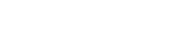##• 類別
數學分析軟體
• 介紹
Berkeley Madonna is an incredibly fast, general purpose differential equations solver. Its graphical interface provides an intuitive platform for constructing complex mathematical models with ease using symbols rather than writing equations. The software provides a suite of graphical tools for plotting your results.
• 價格

Solves:
• • Ordinary Differential Equations
• Difference Equations
• • Multi-dimensional transcendental algebraic equation roots
• • Discrete simulations using conveyors, ovens, and queues

Easy to Use:
• • Type equations directly into equation window in ordinary mathematical notation.
• Click Run. Solutions are automatically plotted. Buttons on toolbar allow variables to be toggled on and off the graph.

Special Interfaces:
• • Flowchart Editor - create models visually with icons and let Berkeley Madonna write the equations.
• • Chemical Reactions - write chemical equations using conventional chemical notation. Berkeley Madonna will automatically apply the appropriate rate law (e.g., mass action) and generate kinetic equations for you.

Very Fast Execution:
• • Berkeley Madonna's impressive speed makes it suitable for large-scale systems, stochastic models, curve fitting, root finding, batch processes, parameter plots, stiff systems, etc.

Parameter Exploration:
• • Change parameter values directly using the parameter window.
• •  Parameter Sliders - move the slider and the model runs instantly and displays the new solution.
•  Automatic scan of Parameter Space - define a range for a parameter and Berkeley Madonna computes and plots a family of curves spanning the range.
• • Parameter Plots - select an attribute (min, max, mean, frequency, etc.) of any variable. Berkeley Madonna automatically plots the attribute as a function of a parameter.
• Sensitivity Analysis - plots the partial derivative of any variable with respect to any parameter.
• • Optimization - searches the parameter space for a point that minimizes an arbitrary expression.

Integration Algorithms:
• • Euler (1st order)
• •  Runge-Kutta (2nd and 4th order)
•  Adaptive stepsize (4th order Runge-Kutta)
• • Stiff ODE solver (Rosenbrock)
• • Custom DT - write your own equations for adjusting stepsize. Allows for stochastic modeling using methods such as the Gillespie algorithm.

Import Experimental Data:
• • Curve Fitter - estimate parameters by fitting solution to one or more imported data sets.

Other Capabilities:
• •  Fast Fourier Transform - plot results in frequency domain.
• •  Array notation (dimensioned variables)
•  Hybrid multi-dimensional root solver used to automatically set up steady-state initial conditions. Can also be embedded in integration loops.Intuitive interface
• Select various icons from the toolbar to quickly build models, while the equations are written automatically. Clicking run instantly solves the equations and plots the results.

Excellent visualization
• Results are automatically graphed, and the user can create sliders to quickly explore the influence of changing parameters. Additional tools such as parameter plot and the fast Fourier transform button provide additional graphical insight.
•
Fast execution & more
• Choose from many integration schemes to solve ODEs, difference equations, and discrete simulations. Easily extract parameter estimates from data with the curve fitting interface, and plot results for a sweep of parameter values with the batch runs - just a few of Berkeley Madonna's many features.
•Berkeley Madonna is an incredibly fast, general purpose differential equations solver. Its graphical interface provides an intuitive platform for constructing complex mathematical models with ease using symbols rather than writing equations. The software provides a suite of graphical tools for plotting your results.### Maxima 5.43 數學符號運算專家系統

Maxima是一種用LISP编寫的電腦代數系統（Computer Algebra System），用於公式推導與符號計算### MOSEK 9.1 數學分析軟體

MOSEK Optimization Tools :大規模級別數學優化軟體  MOSEK Optimization Tools 是一款用來解決大規模級別數學優化問題的軟體。 MOSEK提供了特定解決線性編程、混合整數編程以及其他非線性轉換優化問題。